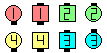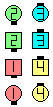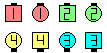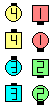Definitions of Square Dance Calls and Concepts

Index -->  Plus  |  A1  |  A2  |  C1  |  C2  |  C3A  |  C3B  |  C4  |  NOL  |
Definitions (Text Only) -->  Plus  |  A1  |  A2  |  C1  |  C2  |  C3A  |  C3B  |  C4  |  NOL  |
 Find call:

\$BJ?9T\$J(B Line \$B\$+\$i(B.

\$B30\$r8~\$\$\$?%+%C%W%k(B (\$B\$^\$?\$O;X<(\$5\$l\$??M(B) \$B\$O(B Cross Fire \$B\$N<+J,\$N%Q!<%H\$r9T\$\$(B, \$BB>\$N?M\$O(B Vertical Tag The Line \$B\$r9T\$\$\$^\$9(B.

Column \$B\$G=*\$o\$j\$^\$9(B.Gee Whiz\$B\$NA0(B \$B8e(B

\$BCm
• Gee Whiz \$B\$N%*%j%8%J%k\$NDj5A\$G\$O(B lead Couple \$B\$NF0\$-\$r(B 1/2 Wheel Across, Step Thru, Face In \$B\$H5-=R\$7\$F\$\$\$^\$9(B. \$B;dC#\$NDj5A\$G\$O(B Cross Fire \$B\$r;H\$C\$F4J7i\$K\$7\$F\$\$\$^\$9(B.
• \$B30\$r8~\$\$\$?(B Line \$B\$+\$i(B, \$B\$I\$A\$i\$N%+%C%W%k\$,(B Cross Fire \$B\$r\$9\$k\$+\$O%3!<%i!<\$,;XL>\$9\$k\$G\$7\$g\$&(B (\$BNc\$(\$P(B, 'Right Gee Whiz' \$B\$O(B \$B1&B&\$N%+%C%W%k(B \$B\$,(B Cross Fire \$B\$r\$7(B, \$B:8B&\$N%+%C%W%k(B \$B\$O(B Vertical Tag The Line \$B\$r\$7\$^\$9(B). \$B\$b\$7%3!<%i!<\$,(B 'No one Gee Whiz' \$B\$H8@\$C\$?\$H\$-\$O(B, \$BA40w\$G(B Vertical Tag The Line \$B\$r\$7\$^\$9(B. \$B\$b\$7%3!<%i!<\$,C/\$b;XL>\$7\$J\$+\$C\$?\$+(B, \$B\$^\$?\$O(B 'Everyone Gee Whiz' \$B\$H8@\$C\$?\$H\$-\$O(B, \$BA40w\$G(B Cross Fire \$B\$r\$7\$^\$9(B (Double Pass Thru formation \$B\$G=*\$o\$j\$^\$9(B). Right Gee Whiz \$B\$O(B \$B:8 \$B\$G=*\$o\$j(B, Left Gee Whiz \$B\$O(B \$B1& \$B\$G=*\$o\$k\$3\$H\$KCm0U\$7\$F\$/\$@\$5\$\$(B.
•Left Gee Whiz\$B\$NA0(B \$B8e(BChoreography for Gee WhizComments? Questions? Suggestions?

https://www.ceder.net/def/geewhiz.php?language=japan&level=C3B
22-March-2023 03:20:23